# AP Computer Science A : Trees

## Example Questions

### Example Question #71 : Standard Data Structures

On a standard binary tree, what would the data structure look like if we inserted the following names into the tree, supposing that names are compared in a standard lexicographic order:

Isaac, Henrietta, Nigel, Buford, Jethro, Cletus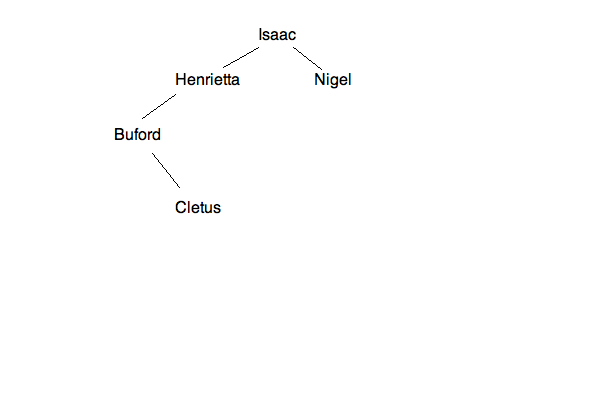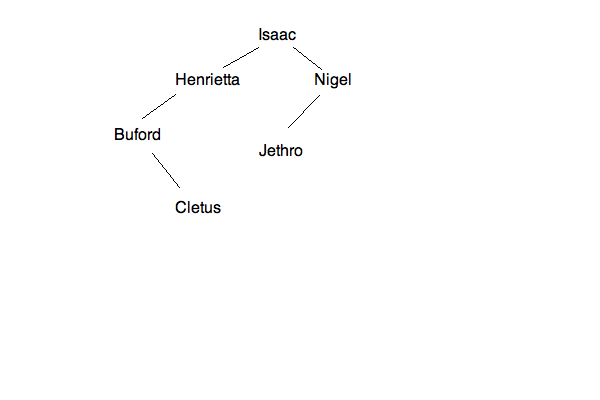None of the trees are correct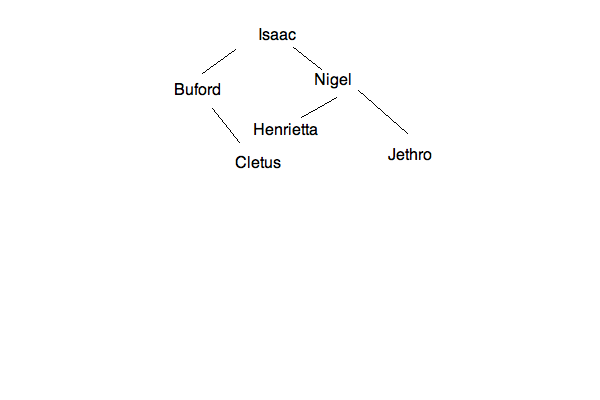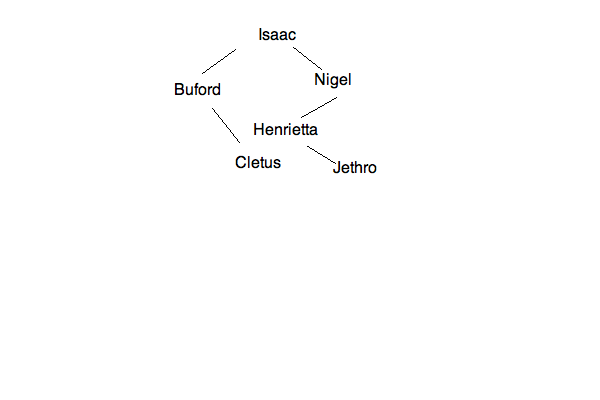Explanation:

A standard Binary Tree inserts into the root first.  It then tries to insert to the "left" for values that are smaller and to the "right" for values that are larger.  Therefore, for the data given, we have the first step: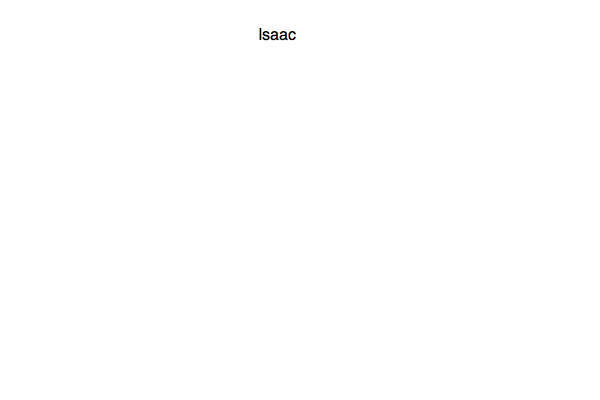Next, you will insert "Henrietta" to the left, for that is less than "Isaac":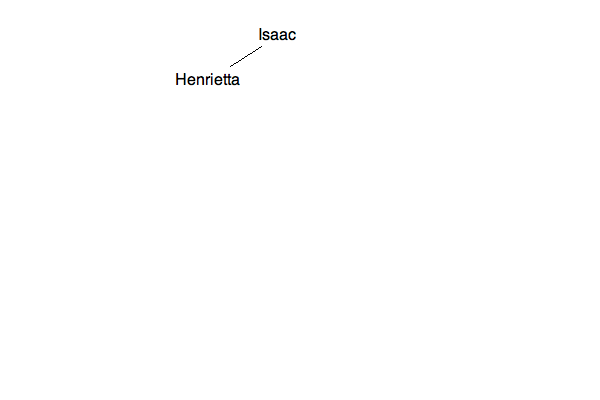Next, "Nigel" is greater than "Isaac":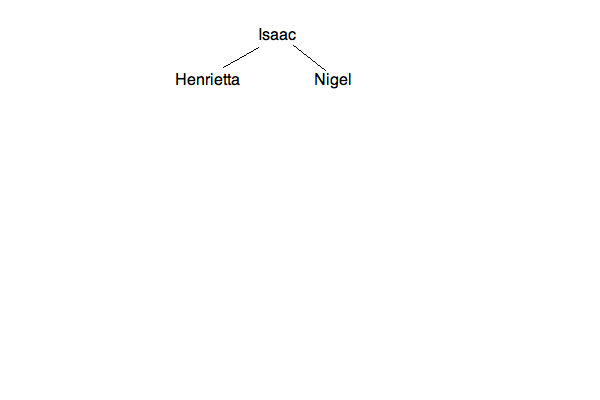Then, "Buford" is less than "Isaac" and then less than "Henrietta":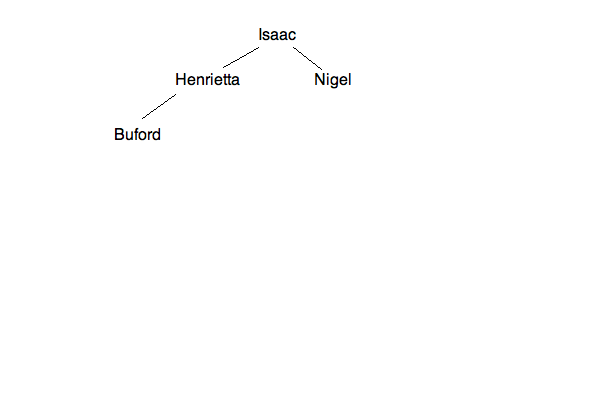This continues through the following stages: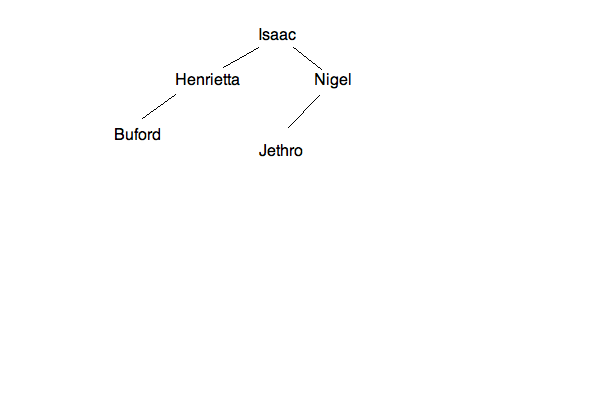Thus, the last image is your final tree!

## POST-ORDER TRAVERSAL

GIVEN THE FOLLOWING TREE: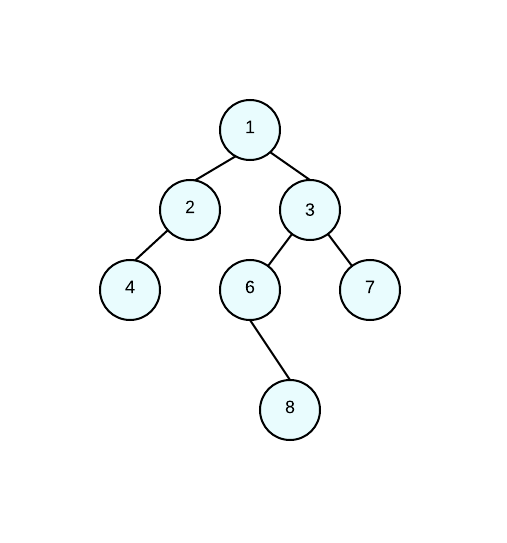WHAT IS THE RESULT OF A POST ORDER TRAVERSAL?

POST ORDER TRAVERSAL: 1, 2, 3, 4, 6, 7, 8

POST ORDER TRAVERSAL: 1, 2, 3, 4, 6, 7, 8

POST ORDER TRAVERSAL: 8, 7, 6, 4, 3, 2, 1

POST ORDER TRAVERSAL: 4, 2, 8, 6, 7, 3, 1

POST ORDER TRAVERSAL: 4, 2, 8, 6, 7, 3, 1

Explanation:

When doing a post-order traversal we go in the following order:

left, right, root.

This means that we are doing any left nodes first, then the right nodes, and LASTLY, the root nodes. If a node is a parent, then we must go throught the left and right children first. Since we're doing post-order traversal, the main root is going to be LAST.

In our example, 1 is a parent so we go to it's left child who is also a parent to node 4. This means that 4 is our first number in the traversal.

POST ORDER TRAVERSAL: 4

Since node 2 doesn't have a right child, we then make that node our next number in the traversal since node 2 it's the left child of node 1.

POST ORDER TRAVERSAL: 4, 2

By now, we have traversed the left nodes of the root. Now we move on to the right subtrees of node 1. Since node 3 is a parent node, we go to it's left child first (node 6). Since node 6 is also a parent, we move on to its children. Node 6 doesn't have a left child. Therefore our next number in the traversal is its right child (node 8) and then the subtree's root (node 6).

POST ORDER TRAVERSAL: 4, 2, 8, 6

Now, we've traversed the left children of node 3 we need to traverse the right child (node 7) who doesn't have any children of its own.

POST ORDER TRAVERSAL: 4, 2, 8, 6, 7

Lastly since we traversed it's right child, we move to the parent and traverse node 3 and our main root (node 1).

POST ORDER TRAVERSAL: 4, 2, 8, 6, 7, 3, 1